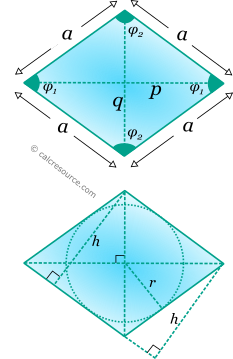## Properties of a Rhombus

This tool calculates the basic geometric properties of a rhombus (also called diamond shape). Enter below the shape dimensions. The calculated results will have the same units as your input. Please use consistent units for any input.

 Known data: Sides and angle Diagonals Geometric properties: Area = Perimeter = Lengths: Side α = Diagonal p = Diagonal q = Height h = Angles : deg rad φ1 = φ2 = Inscribed circle: Radius r =## Definitions

### Geometry

Rhombus (also called diamond shape) is a quadrilateral shape with all four sides equal. Pairs of opposite sides are parallel and pairs of opposite angles are equal. Therefore rhombus is also a parallelogram and features all parallelogram properties. It differs from square in its interior angles which are not all equal and 90°.

The area of a rhombus is given by the formulas:

where a the length of the sides and h the height, perpendicular to a side from an opposite vertex. Height h can be found, using any of the right triangles, with hypotenuse α shown in figure below: